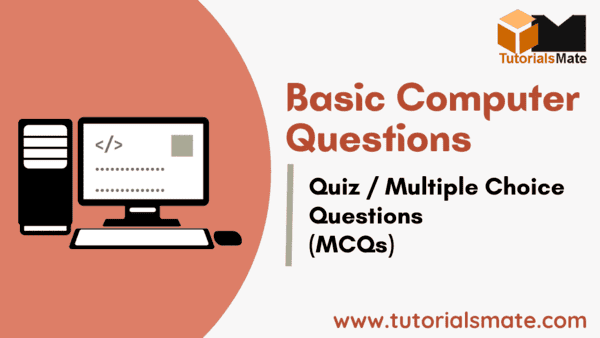# 50 Basic Computer Knowledge Questions AnswersBasic computer knowledge is very crucial in the present time. It is one of the most important sections in almost every competitive exam or online test. We have arranged the basic computer questions that will help you to test your fundamentals. After taking this quiz, you will definitely increase your skills and knowledge about computer systems and its working.

## Basic Computer Knowledge Questions / Quiz Details

 Test Name Computer Basic Questions/Quiz Exam Category Multiple Choice Questions Exam Type Online No. of Questions 50 Questions

So, check out the list of questions below and start taking this quiz:

1.
Which of the following options is true with respect to computers?

2.
Who is known as the father of computer?

3.
Who is credited with the creation of the first mechanical computer, the Difference Engine?

4.
What is the brain of a computer system called?

5.
What does CPU stand for?

6.
Which of the following is included in computer system?

7.
What is known as temporary memory or volatile memory?

8.
What does ALU stand for in the context of computers?

9.
What does RAM stand for?

10.
Which of the following is the smallest unit of memory?

11.
What is Half Byte called?

12.
Which of the following is the primary function of an input device?

13.
Which of the following is not an input device?

14.
Which of the following computer languages is written in binary form?

15.
Which of the following is responsible for performing mathematical calculations?

16.
Which of the following is not a valid function of an output device?

17.
Which of the following is not an output device?

18.
What does SSD stand for?

19.
How many generations are computers currently classified into?

20.
Which of the following is not related to computers?

21.
The term Pentium is related to which of the following computer parts:

22.
Which of the following Indian institutes is credited with the creation of the supercomputer named Pratham?

23.
Which of the following is known as the first mechanical calculating device?

24.
Which of the following operations can be performed through Abacus?

25.
Pascaline is known as:

26.
The first electronic computer ENIVAC (Electronic Numerical Integrator and Computer) was designed by:

27.
Which of the following is known as the first programmable general-purpose electronic digital computer?

28.
Which of the following is known as the first commercially produced digital computer?

29.
Which of the following technology or component was used in second-generation computers?

30.
Which of the following technology or component was used in second-generation computers?

31.
Which of the following generation computers used Integrated Circuits (ICs) as core component?

32.
Integrated circuits used in computers were developed using which of the following materials:

33.
Which of the following generation computers used the microprocessor as core component?

34.
Which of the following options represents a non-volatile memory?

35.
What is meant by GUI in computers?

36.
The data or an instruction given to a computer system is particularly called:

37.
The time taken by a computer to process a specific task is called:

38.
Which of the following options does not represent application software?

39.
Who is credited with the creation of the first supercomputer?

40.
Which of the following OS belongs to IBM?

41.
What was the name of the first microprocessor introduced by Intel?

42.
Which of the following files stores Windows settings?

43.
Which of the following non-volatile memory can be written only once?

44.
A megabyte, also known as a MB, contains:

45.
What does EEPROM mean?

46.
What is the role of computer ports?

47.
Which of the following options is true?

48.
Which of the following cannot be used as a part of secondary storage in a computer?

49.
The collection of 8 bits makes:

50.
When we press a key on the keyboard, the computer system uses which of the following standards to convert the keystrokes into corresponding bits?